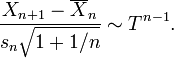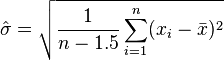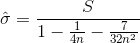When we don’t know the parameter of a population we have to use our sample data to predict the parameter.

Frequentists confidence interval

Let us assume we know that the population mean is mu and standard deviation is sigma.

if we take all possible samples from the population – according to central limit theorem –

95.4% of sample means will end up to be within the range of -2sigma/sqrt(n) and +2sigma/sqrt(n) around mu.

the Xbar will be within -2sigma/sqrt(n) and +2sigma/sqrt(n) far from the mu 95.4% of the time.

This means when we get an Xbar from our sample, there is 95.4% chance that this specific Xbar is within -2sigma/sqrt(n) and +2sigma/sqrt(n) far from the mu.

this mean that 95% of the time the range of -2sigma/sqrt(n) and +2sigma/sqrt(n) around xbar covers mu.

============================================================================

Prediction interval

is the probability of an event in the population to happen based on the sample statistics.

the best estimate for mu is xbar.

the best estimate for sigma we should use to estimate the next event based on the past events is Sx*(sqrt(n+1/n)) with a T distribution of n-1 dfthis shown to be T

http://en.wikipedia.org/wiki/Prediction_interval#Unknown_mean.2C_unknown_variance

for n=10

sigma=s*1.049 but we have to go to row 9 of T table and go 2.262sigma around the mean  2.3724s

which is more than using unbiased estimator for sigma and using normal.

If we use the Unbiased rule-of-thumb estimation of standard deviation=if the above is T then we must use t distribution here for prediction

\hat{\sigma}=\sqrt{\frac{2(n-1)}{2n-3}}S_x

sigma=s*1.029 and we go to 2.262 sigma around the mean 2.328s

or

if we use the Unbiased estimation of standard deviationthen use T distribution for prediction

http://en.wikipedia.org/wiki/Unbiased_estimation_of_standard_deviation

for n=10

sigma=s*1.0274 and we go to 2.262 sigma around the mean which is 2.324s

============================================================================

Credible interval

Let us assume that we don’t know anything about population and we take a sample with a mean of Xbar.

One can claim that mu is closer than Xbar-2sigma/sqrt(n).

if we accept that as H.

P(closer than O|H) is 47.6%.

P(closer than O|H)=P(closer than O|H)*(P(H)/P(O)

but for us, who don’t know anything about that population P(H) and P(O) are the same.

Therefore

P(closer than O|H)=P(closer than O|H)

In this case with no prior information confidence interval and credible interval are the same.

=============================================================================

http://www.webapps.cee.vt.edu/ewr/environmental/teach/smprimer/intervals/interval.html

Since 11 April 2023: 191 total views,  1 views today